www.RomanBlack.com

The Black RNG Engine
My algorithm for generating good quality random data - Orig Jan 2008, Re-write and update; 25 Nov to 6th Dec 2010.

NEW! I have now released my Windows BlackRNG software v1.0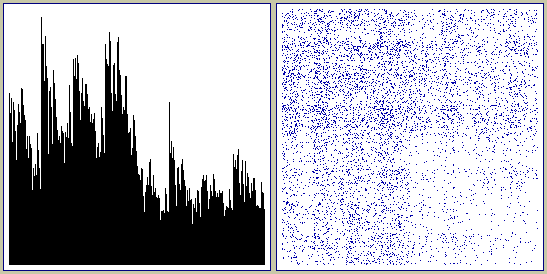What is it?

This is a simple algorithm that is performed by a computer to generate a stream of high quality "random" data.

The Black RNG algorithm can be used to generate finite amounts of random data or can form part of a hybrid RNG to produce streaming data with no limitation on amount.

Benefits of this RNG

1. It can have an extremely large number of max_states with little to no speed penalty.

2. It requires only simple bitwise and indexing operations, no multiply or divide. This makes it feasible on many platforms including microcontrollers.

3. It is chaotic with 2 separate large random systems (cache and engine) that work to constantly scramble each other.

4. It generates high quality entropy and is extremely tolerant of poor seeding or re-seeding due to the "pattern smashing" nature of the RNG and very large number of states.

5. It can generate data quite fast, better than 1 output bit per 100 assembler instructions (if optimised).

6. It has no calculatable fixed period, only a rising probability of repeat after enough iterations.

7. It toggles cache bits, assuring the cache always approaches equal bit probability of 1's and 0's.

8. It transposes bits throughout the cache, assuring seeding or partial re-seeding is eventually distributed throughout the entire cache.

9. It only processes a small percentage of the cache with each pass, so bits in the cache are of different ages and have been scrambled by different engine configurations and a different amount of times.

10. It has characteristics that make short-term synchronous repeats either impossible or very unlikely.

11. Being tolerant of poor seeding it is ideal as part of a hybrid RNG where it is partially re-seeded by a low but natural entropy source.

12. There is no requirement for any specific cache or engine size, giving a very large range of possible configurations to reduce reverse engineering.

13. The chaotic nature of the RNG where cache bits have been scrambled in different ways makes it infeasible to reverse engineer the RNG from observing its output alone. (The "black box" test).

14. It cannot be run in reverse due to some of its state data being destroyed over time, possibly qualifying it under the CSPRNG tests.

Limitations

In its base configuration this is a psuedo random number generator because for a given starting seed + state it will produce the same sequence of numbers.

Because it uses a scrambling (crypographic) process and not a linear process it has no fixed repeat period that can be calculated.

However it does have a limit to the maximum possible period, which is the maximum number of states. A global repeat of all the output data wil occur at an unknown period ranging somewhere between;
A. Somewhere above zero iterations (see later) with incredibly low odds of occurring.
B. Rising to a guarantee that a global repeat must have occurred by the time iterations = max_states.

An "iteration" is defined by the smallest cyclic event the RNG makes, which is one "engine event" as described in the algorithm section below.

Calculating the maximum number of states

The RNG has a very high number of states, that can easily be increased at the cost of RAM only, with little cost in execution speed.

The number of states can be calculated by;

Cache_permutations is simply 2 to the power of cache_size in bits.

Cylinder_permutations is more complex and depends on the number of cylinders and the values used in the cylinders. Information kindly provided by MisterT of Finland;

```If you use 17+1 cylinders and the possible cylinder values are
these 18 numbers: {1,2,3,1,2,3,4,5,7,1,2,3,6,4,1,3,11,9}

Number of unique cylinder permutations is:

18! / (4! * 3! * 4! * 2!) = 926 269 344 000

You must divide by the factorials because you have number 1 four times in
the possible values, number 2 three times etc. If all the numbers in the engine were
unique, the number of permutations would be 18! / (18-17)!.
In general, if you choose k cylinders from n unique numbers, the number of
permutations is n! / (n-k)!.```

Calculating the max states of my test RNG

This RNG setup was used in many of the tests seen below;
Cylinders = 17+1, cache_size = 8000000 bits, mask_size = 8 bits
Cylinder values = {1,2,3,1,2,3,4,5,7,1,2,3,6,4,1,3,11,9}

max_states = cylinder_permutations * cache_permutations * mask_permutations

max_states = 926269344000 * 2^8000000 * 2^8

max_states = 0.926*10^12 * 1.746*10^2408262 * 2.56*10^2

max_states = 4.139 * 10^2408276

Because the 8 million bit cache equals about 1.7*10^2408262 (yes that is 2.4 million decimal digits!) the total number of max_states is extremely large. (Math currently open to scrutiny)

Failure modes of the RNG

It shares the 2 possible failure modes common to all algorithmic RNGs;
1. Possibility of producing self-perpetuating patterns in the cache.
2. Possibility that a global repeat occurs while producing a finite length data output string.

Patternation.
It is expected that this RNG should have a very high resistance to patternation given a large cache and enough cylinders.

With a large enough cache the engine has been shuffled many times by the time it makes a repeat pass through the same area of the cache. The odds are extremely high that the engine and mask will be different to the last values present on the previous pass, and this means that a different form of scrambling will be performed on the cache data the second time.

There is a further chance that the second pass will be indexed differently based on the engine move value used to initiate the pass. The bit index can be displaced anywhere between the lowest and highest cylinder values. With lowest and highest cylinder values in my example being 1 and 11 there are 10 possible bit indexes used on the follwing pass with greater odds of the bit index being somewhere in the range 1-5.

So in my test engine setup above where the engine has been shuffled 8400+ times since the last pass, the chance of a second pass performing the same scrambling process on that same area of the cache approaches this approximate value;

1 / (cylinder_permutations * mask_permutations * 5)
1 / (0.926*10^12 * 2.56*10^2 * 5)
1 / (11.85*10^14)
1 / 1185000000000000

A second situation exists because some bits are toggled in each pass, so even with an identical state and identical process the cache cannot be the same on a second pass. At least some of the cache (10%-30%) must be inverted from any pass to the next.

This rules out any exact repeat of that same area of the cache at a period of exactly 1*cache_size (a synchronous repeat).

Global repeat.
The RNG will perform a global repeat any time it revisits the same state. Fortunately the number of states is very large, ss seen above in "Calculating the maximum number of states".

Possibility of global repeat with period < cache_size
With a small cache this may be possible, especially with carefully chosen seed and cylinder values to try to force patternation to begin. However it is extremely unlikely as the cache must be divided into equal sized divisions of identical data, identical apart from the exact same bits that will be toggled in one pass.

The most likely chance of this happening is if a very small cache is entirely filled with a short repeating seed, and the engine has a small number of cylinders and a mask match is never found so an engine shuffle never occurs. This can be seen in extremely small compromised versions of the RNG.

In actual use with a large cache this failure would never be expected as the "pattern smashing" nature of the RNG means that a cache consisting entirely of evenly divided patterns of this type is *much* less likely than an entropic state.

One bulletproof fix is to use a cache_size which is a prime number, that cannot be filled with an even number of identical strings. This is recommended when you must use a smaller cache size but may be unneccessary with larger cache sizes.

Possibility of global repeat with period = cache_size
A synchronous global repeat of 1*cache_size is not possible with the RNG as every pass through the cache toggles some bits, so a second pass cannot leave the same cache data as the first pass.

A synchronous global repeat of 2*cache_size is technically possible with small cache and engine sizes where engine shuffling has not occurred during both passes and requires that the exact same bits are processed and the same bits will be toggled 1 on one pass and back to 0 on the next pass.

Once the cache is large enough that significant engine shuffling occurs then any short term synchronous global repeat becomes infeasible as the mask match used for engine shuffling will not occur in the same places in the toggled cache as it does in the non-toggled cache. This means that each of those 2 passes will produce a different sequence of shuffling of the engine values.

Possibility of long term non-synchronous global repeat
This is the only likely global repeat in the RNG assuming proper seeding and a large enough cache and frequent engine shuffles.

A non-synchronous global repeat will occur if the RNG happens to randomly experience the same state that has already occurred at some previous point in it's operation. The maximum theoretical number of states is shown above in "Calculating the maximum number of states".

As the chaotic nature of the RNG seems to generate very high entropy in its cache (see results below) and its cache is used to generate future states it is possible that the probability of global repeat approaches the "best case" which would be calculated from the probability of repeat of any 2 identical states within max_states.

It is expected that the probabiliy of repeat will be higher (worse) than the theoretical best case if the RNG is producing less than perfect entropy within its cache. This becomes more likely if the cache or engine sizes are small or if there are less engine shuffles occurring.

The Black RNG algorithm

This is how the engine processes the data and is called one "event";

• TRANSPOSE one bit with a bit that was "held".
• TOGGLE (invert) another bit.
• MOVE forward a few bits.
(then repeat)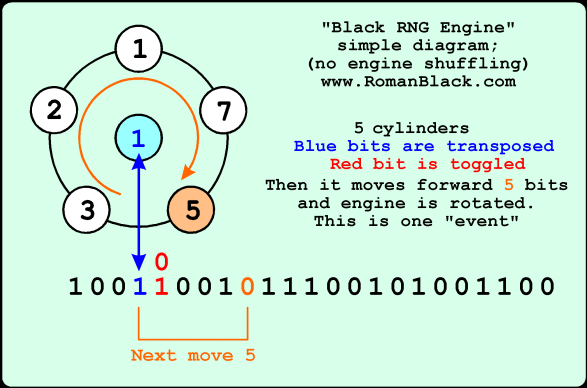The diagram above shows an engine with 5 "cylinders" and one "held" bit. An engine event transposes the (blue) bits so the held bit is swapped with the bit in the cache. The engine event also toggles the next (red) bit. Then the engine moves forward 5 bits ready to repeat. The engine cylinders are rotated one place, so the next move distance will be 7 bits.

The engine will skip through the cache bits, processing some bits but not all. Over multiple passes there will be a very complex beat frequency of the cylinder values vs the length of the cache that scrambles the cache bits quite well but still in a complex pattern.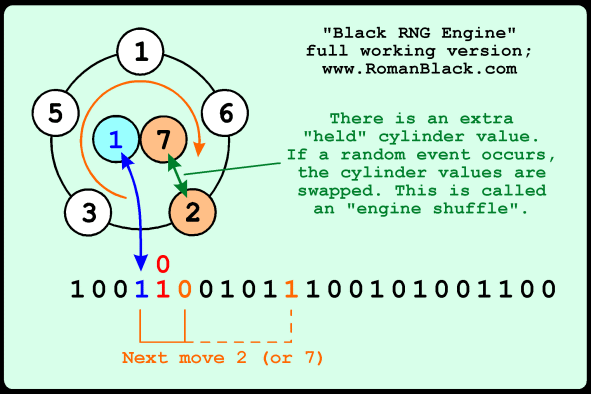Above shows the same basic system, but with one addition. There is now a "held" cylinder value, as well as a held bit. When a random event is detected the cylinder values are swapped, this "engine shuffle" means that the engine has many possible configurations, and each engine configuration will scramble the cache bits with a different complex pattern.

If the engine is shuffled many times per pass through the cache then eventually any cache bit will have been processed by different configurations of the engine, at different times, and an unknown amount of times.

Also, as bits are picked up and put down in different locations bits will be transposed from areas of the cache that may not have been affected for many passes to areas that are being affected by passes now. Combined with the fact that the engine configuration changes over time this is scrambling new bits together with old bits that were previously scrambled by a vastly different engine configuration.

Originally I called this; "Transposing bits in both space AND time" and it is instrumental in generating the high level of chaos in this RNG.

The "random event" that causes the cylinder value swap can be anything of acceptable frequency including a real-world stimulus. For a self contained RNG I used a bitmask (typically 8 bits) compared to the last 8 bits that have been transposed. So on average, an engine shuffle will occur after every 256 engine events.

The Black RNG algorithm (actual implementation);
1. Transpose the held bit with current cache bit
1b. Shift a copy of the new held bit into the 8bit mask test
2. Toggle the bit after the current bit
3. Using X value from engine, move forward in cache, X bits
4. Rotate engine to next cylinder
5. Test the mask, see if it matches the last 8 transposed bits
5b. If it matches; swap the current cylinder value with held cylinder
(repeat)

Details of the implementation in C code

For convenience of indexing the bits in the cache, I just used 1 byte to represent each bit. This costs RAM, 8 times more, but for a PC based implementation RAM is usually plentiful and there is a speed and simplicity payoff for being able to address any cache bit with a single index number. All bytes in the cache are either 0x01 or 0x00 and each byte represents one cache bit.

The cache should be filled with a seed, this was accomplished by loading a file and repeating it throughout the cache until the cache is full (or using a file >= cache size to fill the cache). Generally a text file was used.

The C/C++ code below was just copied directly from my Windows software with no attempt to tidy it up. It is a bit clunky but is fully workable as a Black RNG Algortihm that you should be able to adapt to your own C software. No payment is required but please mention me as the author of the code and Black RNG algorithm.

``````
//===========================================================================
//  GENERATE_ENTROPY    (Black RNG algorithm - see www.RomanBlack.com)
//===========================================================================
void __fastcall generate_entropy(void)
{
long i=0;
long e=0;

long pos=0;
long next_pos=0;
long engine_pos=0;
long engine_last_pos=0;

unsigned char engine_value=0;
unsigned char engine_last_value=0;

long shuffle_count=0;

long loops=0;

long bar_count=0;

unsigned char c=0;
unsigned char bit=0;
unsigned char last_bit=0;

unsigned char astring;           // for reading Edit box!

signed long max_loops=0;

//--------------------------------------------------
// This generates 1Mb of entropy data from the
// 1Mbit seed data;
//  starts with 1Mb seed already in seed_data[]
//  copies into 8Mb in ent_data[] (this is the working "cache")
//  performs the scrambling in ent_data[]
//  ends with 8Mb in ent_data[] (1byte=1bit)
// Note! for indexing convenience the engine uses 1byte for each cache bit

//--------------------------------------------------
// clear progress bar
Form1->ProgressBar1->Position = 0;

//--------------------------------------------------
// first clean ent_data[]
for(i=0; i<8000000; i++)     ent_data[i] = NULL;

//--------------------------------------------------
// copy 1Mb seed_data[] into 8Mb in ent_data[]
e=0;
for(i=0; i<1000000; i++)
{
// get the char
c = seed_data[i];

// if bit in c is set, then ent_data byte=1;
if( c & 0x80 )    ent_data[e+0] = 1;
if( c & 0x40 )    ent_data[e+1] = 1;
if( c & 0x20 )    ent_data[e+2] = 1;
if( c & 0x10 )    ent_data[e+3] = 1;

if( c & 0x08 )    ent_data[e+4] = 1;
if( c & 0x04 )    ent_data[e+5] = 1;
if( c & 0x02 )    ent_data[e+6] = 1;
if( c & 0x01 )    ent_data[e+7] = 1;

// move to next pos (next 8 bytes) in ent_data
e += 8;
}

//--------------------------------------------------
// next do the scrambling!!
//--------------------------------------------------
// Black RNG scramble procedure;
//  1. exchange the picked up bit at current pos
//    1b. shift that new bit into mask (ie keep record of last 8 bits)
//  2. toggle the following bit
//  3. using X value from engine, move forward X bits
//  4. inc engine one cylinder
//  5. check last 8 exchanged bytes, if == mask;  (1 chance in 256)
//    5a. exchange engine value (shuffles engine)
//  Repeat (until scrambled enough)
//--------------------------------------------------

#define ENG_SIZE 17    // number of cylinders

// load the engine with our 17 cylinder values (refine these later after testing?)
engine_last_value=9;    // held value at the start, ready to exchange

engine = 1;     // these values work well enough
engine = 2;
engine = 3;
engine = 1;
engine = 2;

engine = 3;
engine = 4;
engine = 5;
engine = 7;
engine = 1;

engine = 2;
engine = 3;
engine = 6;
engine = 4;
engine = 1;

engine = 3;
engine = 11;    // one larger prime value breaks up patterns

//--------------------------------------------------
// prepare to loop and scramble the data
loops = 0;
bit = 0;
last_bit = 0;
shuffle_count = 0;

// allows user to change max number of loops on main form!
// must read the edit box and convert to a number...
long length = Form1->Edit1->GetTextLen();     // get length
Form1->Edit1->GetTextBuf(astring,(length+1));   // get text, add 1 for NULL
tstring[length] = NULL;                         // add the null
max_loops =  atol(astring);                     // get as number

if(max_loops > 500)
{
max_loops = 500;
Form1->Edit1->Text = "500";
Form1->Edit1->Repaint();
}
if(max_loops < 1)
{
max_loops =1;
Form1->Edit1->Text = "1";
Form1->Edit1->Repaint();
}

// set up progress bar
Form1->ProgressBar1->Max = max_loops;

// loop and scramble the data
while(loops < max_loops)
{

//  1. exchange the picked up bit at current pos
bit = ent_data[pos];          // pick up new bit
ent_data[pos] = last_bit;     // drop old bit
last_bit = bit;               // save bit for next exchange

//  1b. shift that new bit into mask (ie keep last 8 bits)
if(bit != 0) mask += 0x01;    // put in the new bit

//  2. toggle the following bit
next_pos = (pos + 1);                  // get pos of the next bit
if(next_pos == 8000000) next_pos=0;    // handle cache wrap around

if(ent_data[next_pos] == 0)   ent_data[next_pos]=1;   // now toggle it
else                          ent_data[next_pos]=0;

//  3. using X value from engine cylinder, move forward X bits
pos += engine[engine_pos];    // move X bits forward
if(pos >= 8000000)            // handle wrap
{
pos = (pos - 8000000);     // loop pos
loops++;                   // count 1 more cache loop done!

// also handle progress bar updating here
// update progress bar every 5 loops
bar_count++;
if(bar_count >= 5)
{
Form1->ProgressBar1->Position = loops;
bar_count = 0;
}
}

//  4. inc engine one more cylinder, loop if needed
engine_pos++;
if(engine_pos >= ENG_SIZE) engine_pos=0;

//  5. check last 8 exchanged bytes; if == mask;  (1 chance in 256) then;
//    5a. exchange engine value (shuffles engine)
{
// shuffle (exchange) an engine cylinder value
engine_value = engine[engine_pos];       // save a copy of the current cylinder
engine[engine_pos] = engine_last_value;  // held cyl -> current cyl
engine_last_value = engine_value;        // current cyl -> held cyl

shuffle_count++;       // count how many times engine cylinders have been shuffled
}
}

//--------------------------------------------------
// all done!
Form1->ProgressBar1->Position = Form1->ProgressBar1->Max;

sprintf(tstring,"Loops: %ld  Engine shuffles: %ld",
loops,shuffle_count);
Form1->Label3->Caption = tstring;   // display stats

}
//---------------------------------------------------------------------------

``````

Fine-tuning the RNG

Here are some basic guidelines for setting up a Black RNG.

The cache size should be quite large. 1 Mbit or 8Mbit is good and at 8Mbit it produces >8000 engine shuffles per pass through the cache with an 8bit test mask.

If using a smaller cache, use a smaller test mask (ie 6bit or 5bit) to still give plenty of engine shuffles per pass through the cache. Also as discussed above if using a small cache there can possibly be a benefit to using a cache size that is a prime number.

Engine size (number of cylinders) should be a minimum of about 17+1 as used in my test RNG. If the cache must be small to preserve RAM on a smaller implementation, using more cylinders can help increase the max_states. It is possible that using a very large number of cylinders may compromise entropy by allowing the engine itself to patternise. I would keep the number of cylinders in the 15 to 25 range.

As for choosing cylinder values, about half the cylinder values should be small; 1,2,3. For the remainder use medium sizes 4-9 with the majority being odd if you have many cylinders, although I would include one of each value for maximum entropy. Then at least one larger value for every 10 cylinders or so.

Extracting random data from the RNG

All the information given above is for the base implementation of the Black RNG, and all it does is scramble it's own cache.

For applications where you only need a small amount of random data you could just extract a chunk of data from the cache. However this produces some issues. As the RNG is chaotic its main operation is to constantly smash any patterns that appear in its cache. For this reason, if you just extract the entire cache at any point in time this represents a worst case scenario where the cache contents are highly random but the cache is very unlikely to contain a mass of patterns at any one time. It is verging on being "too" random.

This represents a statistical bias, as real random data has a possibility of being a "mass of patterns" (although this is always a very small possibility).

It was always my intention that the output of this RNG is not its cache itself but is bits extracted over time. The RNG and the cache should be viewed as a "resource" that generates entropy so that data can be extracted over time.

The obvious and my "standard" method would be to extract every bit processed, specifically; to extract each transposed bit after each engine event. This works very well and produces a stream of bits with a volume of about 27% of cache size (with my cylinder values) so it generates around 2.1 million very good random bits per pass through a 8000000 bit cache.

As the RNG is good at producing an unknown value in any cache bit at any time, the data extracted in this way more closely resembles data extracted from other entropic sources like physical ones and as less data is extracted per pass through the cache (ie; extract 1 held bit per N engine cycles) this becomes more so.

See lower down the page for ideas on more complex implementations of the RNG.

Test results for the RNG

25th Nov 2010. I downloaded John Walker's ENT.EXE test program which does a battery of tests on data generated by random number generators.

For the random data I just used the value in the Black RNG "cache", this is a worst case scenario as normally you would generate data on the fly as the engine progresses, with the least data extracted over time the higher the entropy. But, even with the worst case scenario the randomness of the data generated by the Black RNG appears very good;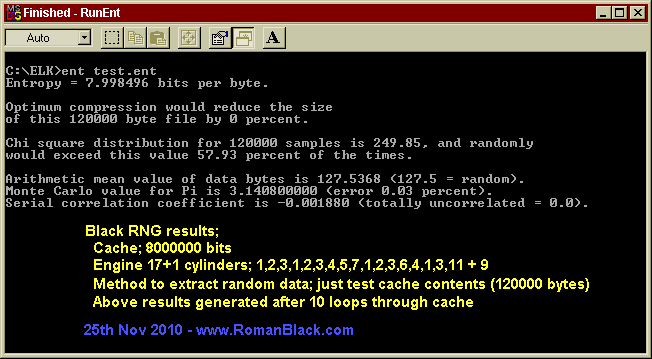```Entropy testing results from Black RNG - 25 Nov 2010

Black RNG engine stats;
seed = just a text file
cache = 8000000 bits
cylinders = 17+1held; 1,2,3,1,2,3,4,5,7,1,2,3,6,4,1,3,11 + 9
method to extract random data; just test the cache contents after X loops

Results generated by John Walker's test software ENT.exe 2008,
see;
http://www.fourmilab.ch/random/

Results of 6 tests of first 120000 bytes of cache contents using the same seed,
after the RNG has done X loops through the cache;

Loops    Entropy;     Compress     CHI square   CHI square   Arithmetic  Monte       Serial
through  (8 perfect)  able         distrib.     percent      Mean        Carlo Pi    Correlation
cache;                percent      (256         (>10 & <90   (127.5      (0 percent  (0 perfect)
(0 perfect)  perfect?)    perfect)     perfect)    perfect)

10       7.998496     0            249.85       57.93        127.5368    0.03       -0.001880
12       7.998601     0            232.34       84.25        127.3019    0.45       -0.002216
30       7.998421     0            262.36       36.23        127.2908    0.06        0.003228
100      7.998554     0            240.86       72.86        127.6494    0.43       -0.003508
200      7.998371     0            270.20       24.52        127.5329    0.25        0.000122
500      7.998429     0            261.70       37.32        127.2676    0.04       -0.000545

My explanation of the test values, much is copied from John Walker's page;

Entropy; The information density of the contents of the file, expressed as a number of bits
per character. A value of 8 means the randomness is so dense (so few patterns) the data cannot
be compressed.

Compressable percent; Same as above.

Chi Square test; The chi-square test is the most commonly used test for the randomness of data,
and is extremely sensitive to errors in pseudorandom sequence generators. If the percentage
is greater than 99% or less than 1%, the sequence is almost certainly not random. If the
percentage is between 99% and 95% or between 1% and 5%, the sequence is suspect.
Percentages between 90% and 95% and 5% and 10% indicate the sequence is almost suspect.
From my limited understanding of statistics math this value will change greatly according
to the actual contents of the data being tested and there is no "perfect" value, but percentages
between 10 and 90 are considered officially statistically "random". (see examples below!)

The low-order 8 bits returned by the standard Unix rand() function, yields;
Chi square distribution for 500000 samples is 0.01, and randomly would exceed this value more
than 99.99 percent of the times.

While an improved generator [Park & Miller] reports;
Chi square distribution for 500000 samples is 212.53, and randomly would exceed this value
97.53 percent of the times.

Chi-square result of a genuine random sequence created by timing radioactive decay events;
Chi square distribution for 500000 samples is 249.51, and randomly would exceed this value
40.98 percent of the times.

Black RNG (after as few as 10 loops, matches performance of a radioactive decay RNG!);
Chi square distribution for 120000 samples is 249.85, and randomly would exceed this value
57.93 percent of the times.

Arithmetic Mean; This is simply the result of summing the all the bytes in the file and
dividing by the file length. If the data is close to random, this should be about 127.5.
If the mean departs from this value, the numbers generated are consistently high or low.

Monte Carlo Pi; Each successive sequence of six bytes is used as 24 bit X and Y co-ordinates
within a square. If the distance of the randomly-generated point is less than the radius of
a circle inscribed within the square, the six-byte sequence is considered a *hit*.
The percentage of hits can be used to calculate the value of Pi. For very large streams
(this approximation converges very slowly), the value will approach the correct value of
Pi if the sequence is close to random. A 500000 byte file created by radioactive decay yielded:
Monte Carlo value for Pi is 3.143580574 (error 0.06 percent).

Serial Correlation Coefficient;
This quantity measures the extent to which each byte in the file depends upon the previous byte.
For random sequences, this value (which can be positive or negative) will, of course,
be close to zero. A non-random byte stream such as a C program will yield a serial correlation
coefficient on the order of 0.5. Wildly predictable data such as uncompressed bitmaps will
exhibit serial correlation coefficients approaching 1.
```

New data from revised windows software

I revised my Windows software to produce histograms (spectral distribution of data) and scattergraphs (data plotted as XY points).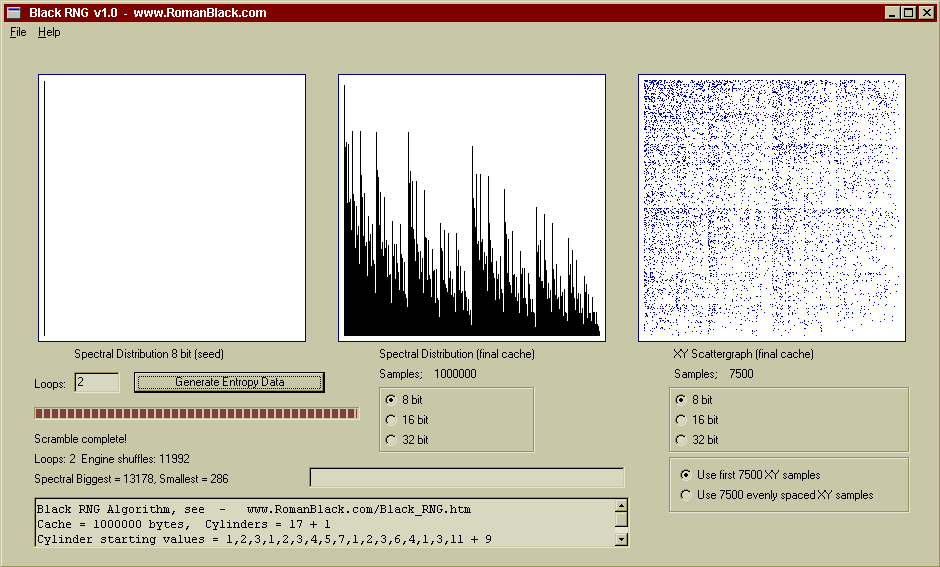Above is a worst case, the seed is blank (all zeros) and after only 2 loops you can see it has stated to randomise the cache although it is nothing like good random data at this point.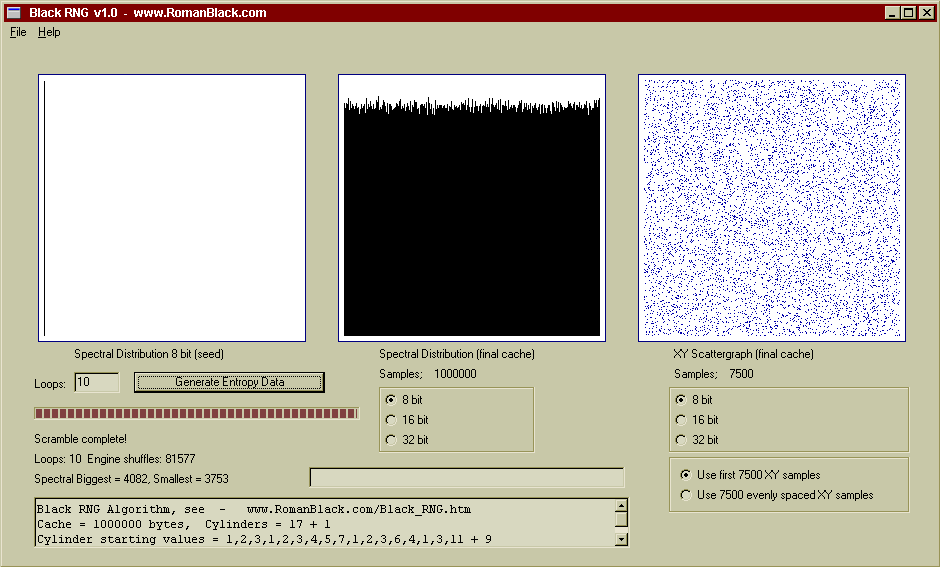Above shows after 10 loops and >80000 engine shuffles it has randomised the cache even with the absence of a good seed.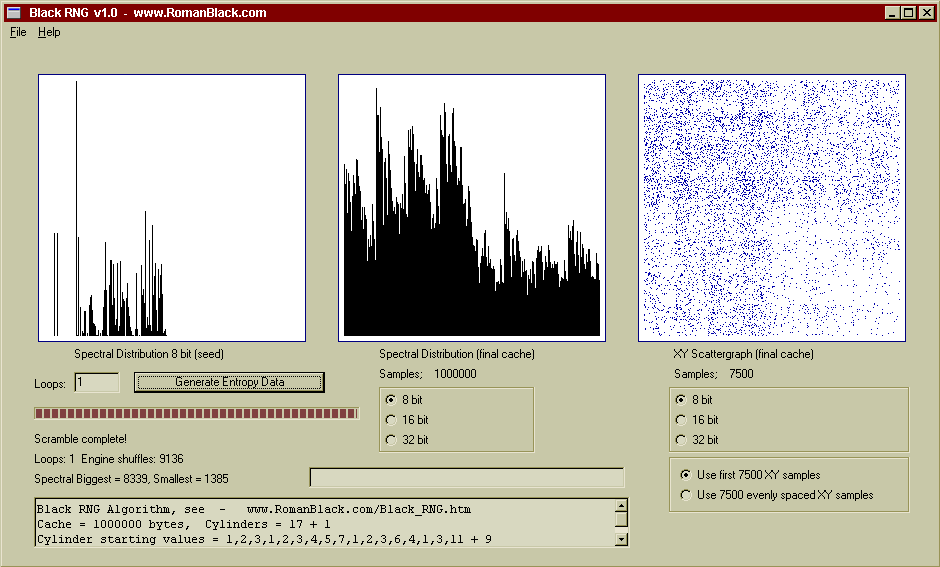Above is a new seed, the same one I used for the "ENT.exe testing" further up the page, it is just a text file. After only one pass through the cache there is some significant randomisation happening.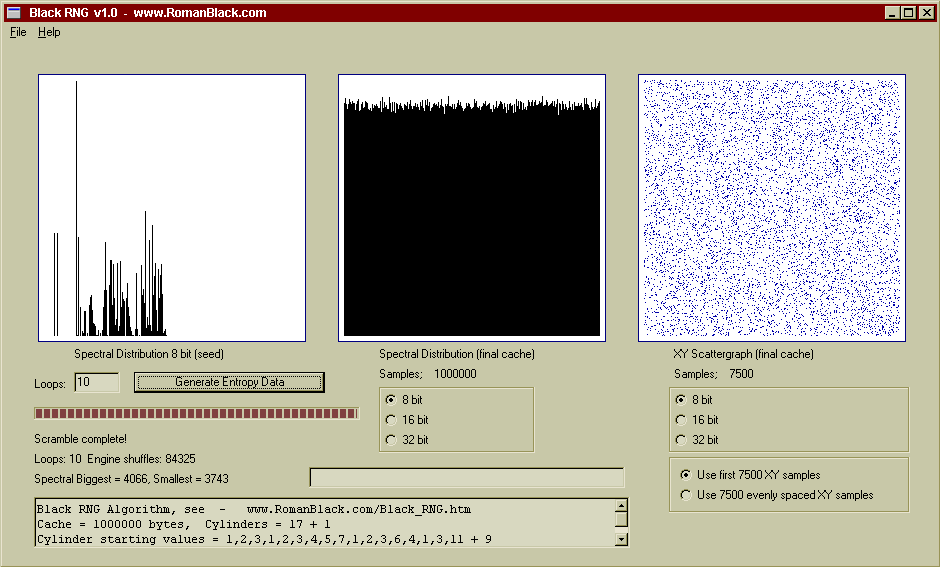Above shows after 10 passes there is very high quality random data. The 1Mb file generated in the above 10 loop test is HERE if you want to do your own entropy testing on it.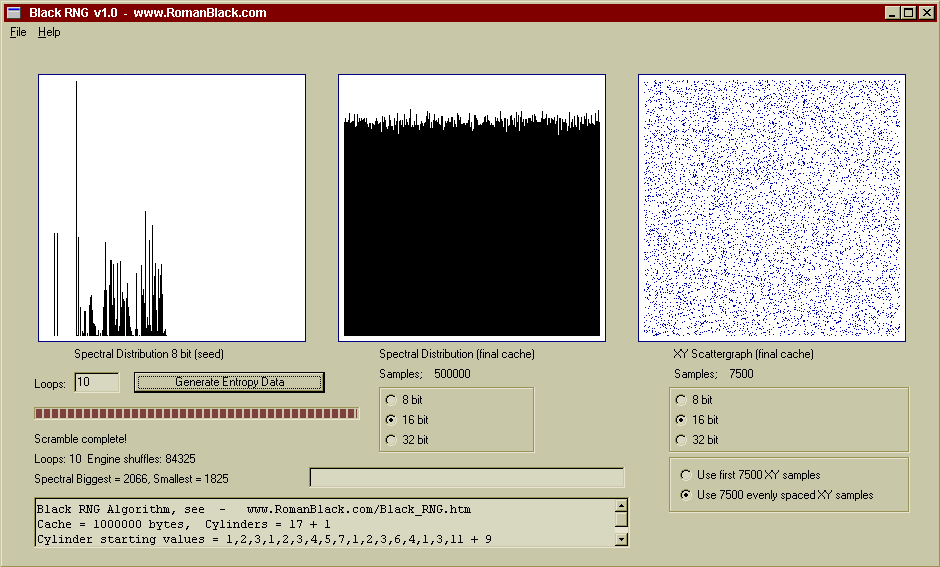Above is the same cache contents, this time displayed as 16bit values. Good random data will look the same whether analysed as 8bit numbers or as 16bit or 32bit numbers. Also this scattergraph was plotted from samples spread throuhout the cache instead of just from the start of the cache. Again, with good random data this will make little difference.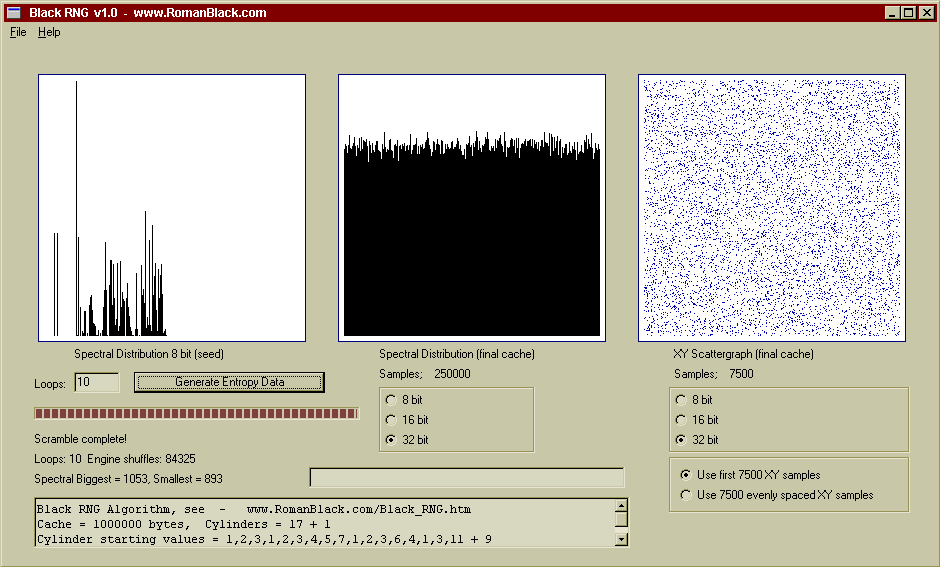Above is the same cache contents, this time displayed as 32bit values. The spectral distribution is as good as with 8bit and 16bit numbers as shown by the histogram, however there are 4 times less samples to graph so the deviation is slightly higher (which is correct).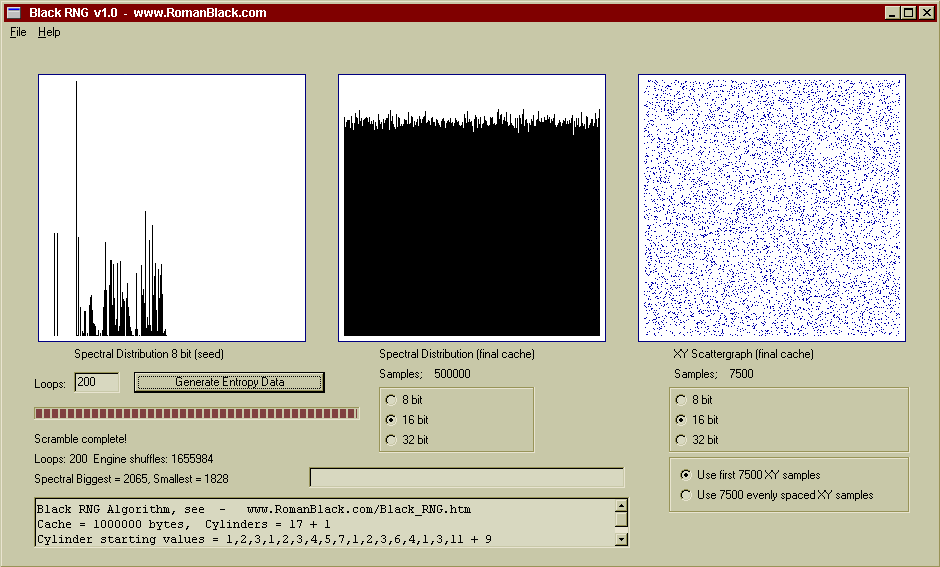Above is a 200 loop test, generated from the same seed. After 200 loops the spectral distribution is still excellent as is the entropy, this is the same 200 loop test as shown above in the ENT.EXE testing.

Testing a smaller version of the RNG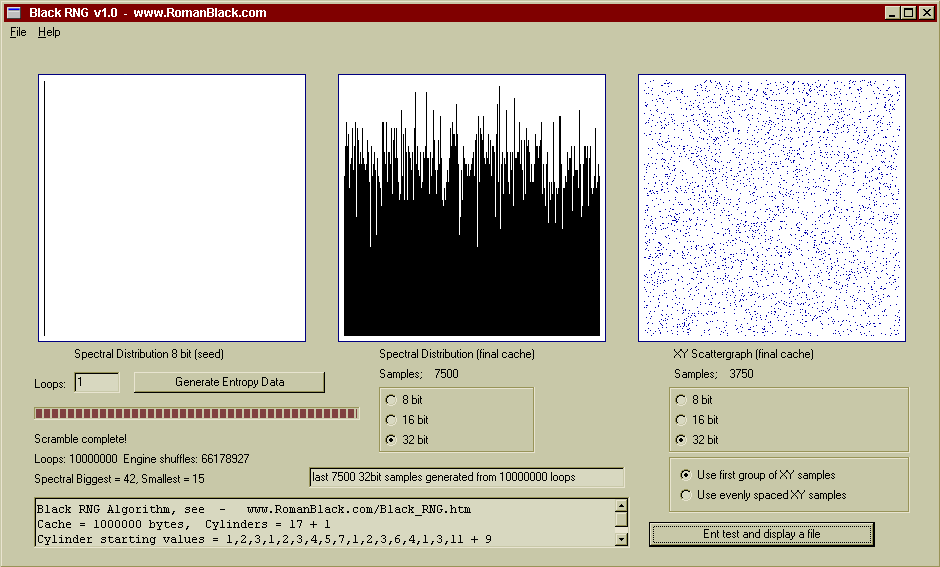Above is a 10 million loop test, performed as a "poor case scenario" in an attempt to get the engine to fail.

RNG setup;
Seed = all zeros!
Cache size = 6400 bits
Cylinders = 17+1 {1,2,3,1,2,3,4,5,7,1,2,3,6,4,1,3,11,9}

Even after 10 million loops the entropy in the cache was still of excellent quality. However this engine is not recommended, it is severely compromised due to these factors;
1. The cache was seeded with all zeros, a poor seed.
2. The cache is too small and is a multiple of 32 bits (data testing was also 32 bits).
3. Due to an 8bit mask there were only 6.6 engine shuffles per pass!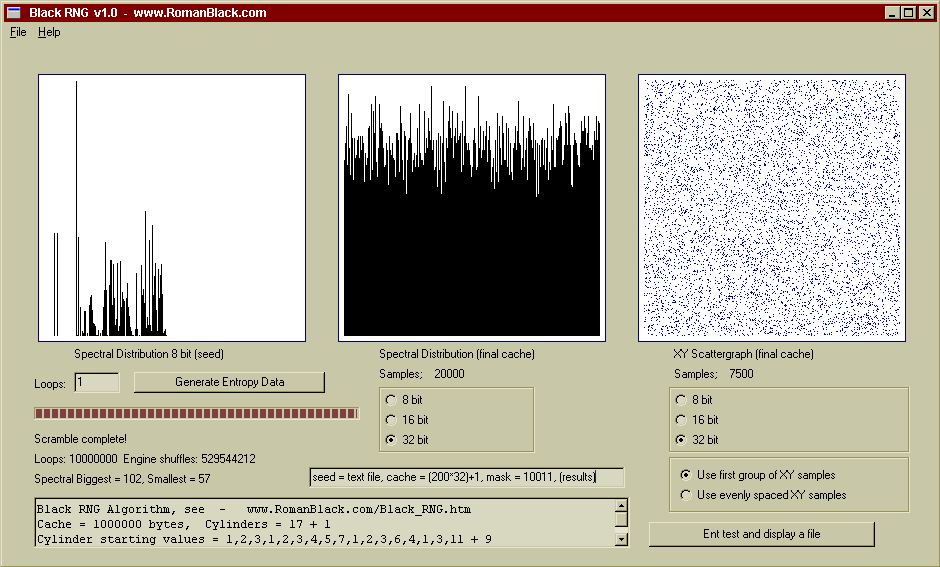Above shows the results from a much improved RNG using a similar sized cache.

RNG setup;
Seed = a text file
Cache size = 6401 bits
Cylinders = 17+1 {1,2,3,1,2,3,4,5,7,1,2,3,6,4,1,3,11,9}

After 10 million loops the entropy in the cache was still of excellent quality. The difference in appearance of data from the 2 engines is due to different sample sizes being plotted. Both produced good cache entropy. However the second engine is superior due to using a cache that is not a multiple of 32 bits (a prime cache size would be even better still) and the important change to a 5 bit mask so that instead of 6.6 engine shuffles per loop the second engine produced 52.9 engine shuffles per loop. It also started with a better seed, although that is less critical.

It appears that smaller Black RNG engines can still produce good entropy although since the cost of a large cache is only RAM with no speed cost it is recommended that a large cache be used if possible.

NEW! 26th Nov 2010 - Black RNG Software

As seen in the above screenshots. So you can do your own tests or generate 1Mbyte of entropic data.

Black RNG v1.0 Software HERE

NEW! 3rd Dec 2010 - PrimeFinder Software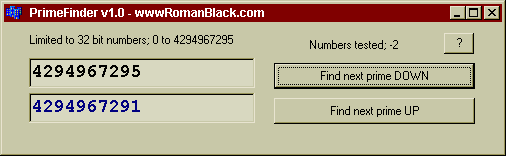I made a simple freeware program to instantly find any prime number in the 32bit range, ie; 0 - 4.29 billion.

It can also tell you if your number is a prime number.

PrimeFinder v1.0 Software HERE

Complex implementations of the RNG?

The chaotic nature of the RNG produces very good entropy that (in the short term) is as good as (or close to) a natural entropy source like a radioactive decay RNG.

However 2 issues still exist;
1. The data is still psuedo-random (sequential) based on the starting seed and the RNG configuration.
2. For generating very large data streams there will be a chance of a global repeat occurring.

Issue 1 will not be a problem for most requirements of generation of a small amount of data, up to some megabytes. This is because the potential number of seeds is so large and the possible number of engine configursations is so large, see "Calculating the max states of my test RNG" above. Changing the cache size from 8000000 bits to 8000001 bits or changing the starting seed by only 1 bit will both give totally different data output sequences, after the RNG has been allowed to re-scramble through enough passes.

Issue 2 should not present a problem when generating a small amount of data due to the large number of states but makes the RNG unsuitable for generating a continuous data stream due to a rising chance of global repeat as time progresses.

If the RNG is used as part of a hybrid RNG, these issues can be avoided. The theory is as follows;
1. A large Black RNG is used for entropy generation.
2. An external system is used to *partially* re-seed the Black RNG with a very small amount of external data, maybe 0.1% of the seed per pass.
3. If required, re-scrambling passes are performed between re-seeding.
4. The constant partial re-seeding means the RNG output sequence changes constantly and also negates any chance of global repeat.
5. The system used for partial re-seeding only needs to meet the requirement that it runs independently from the Black RNG and produces some entropy OR some system for stopping global repeat.

The following Hybrid RNG suggestions are unproven and untested but may be worth exploring.

Period/Black hybrid RNG, fully PC based.
This would use low entropy timed data from the PC clock timer period (which uses its own xtal) that will offer unpredictability when compared against the RNG cache loop that is generated by the PC CPU and CPU xtal. That unpredictability is a low quality entropy but is natural provided the two processes are timed from 2 xtals that are indpendent as each freq is different and differently affected by temperatures within the PC.

Physical/Black hybrid RNG.
This would use any low-ish entropy physical source that is easy to make in hardware, partially re-seeding the RNG. As the physical source does not need to be unbiased or high entropy or fast this allows a lot of options for the hardware attachment. The physical source could be any cheap hardware system like a capacitor charge cycle, zener diode breakdown, neon gas discharge breakdown, audio or RF noise, high resolution sensor readings etc. A simple cheap hardware component could be plugged into a parallel, serial or USB port.

Audio/Black hybrid RNG.
This would use any non-compressed audio music CD. The CD is used to partially re-seed the RNG over time using the most entropic portion of its data; the least significant binary bits of its 16bit stereo audio data. Because the CD holds tens to hundreds of megabytes of good entropy data this should be able to produce a very large but finite amount of entropy free from global repeat. With a re-seed:cache ratio of 0.1% this could produce up to 50 gigabytes of data from a music CD. This system is "almost" self contained within a PC needing only the addition of a CD.

Fractal/Black hybrid RNG.
This would use a simple fractal algorithm process to generate a string of single digit numbers that are guaranteed not to globally repeat because of a known math mechanism. As the RNG will produce the bulk of the entropy the fractal process can be implemented simply with minimal entropy requirements, and good efficiency as the fractal only needs to provide a very small amount of data compared to the output data. Most fractal processes implemented on a computer will have a known repeat period, so this hybrid would be more suitable for generating a known "safe" amount of data than a continual stream. However it would be trivial to produce thousands of gigabytes of Hybrid RNG data even from a small simple fractal.

Is the Black RNG a CSPRNG?

CSPRNG = Cryptographically Secure Psuedo Random Number Generator.

```(Wikipedia;) Requirements of a CSPRNG.
The requirements of an ordinary PRNG are also satisfied by a cryptographically secure
PRNG, but the reverse is not true. CSPRNG requirements fall into two groups:
first, that they pass statistical randomness tests; and
secondly, that they hold up well under serious attack, even when part of their
initial or running state becomes available to an attacker.

* Every CSPRNG should satisfy the "next-bit test". The next-bit test is as
follows: Given the first k bits of a random sequence, there is no polynomial-time
algorithm that can predict the (k+1)th bit with probability of success better than 50%.
Andrew Yao proved in 1982 that a generator passing the next-bit test will pass all
other polynomial-time statistical tests for randomness.

* Every CSPRNG should withstand 'state compromise extensions'. In the event
that part or all of its state has been revealed (or guessed correctly), it should
be impossible to reconstruct the stream of random numbers prior to the revelation.
Additionally, if there is an entropy input while running, it should be infeasible
to use knowledge of the input's state to predict future conditions of the CSPRNG state.

Example: If the CSPRNG under consideration produces output by computing bits of pi
in sequence, starting from some unknown point in the binary expansion, it may well
satisfy the next-bit test and thus be statistically random, as pi appears to be a
random sequence. (This would be guaranteed if pi is a normal number, for example.)
However, this algorithm is not cryptographically secure; an attacker who determines
which bit of pi (i.e. the state of the algorithm) is currently in use will be able
to calculate all preceding bits as well.

Most PRNGs are not suitable for use as CSPRNGs and will fail on both counts. First,
while most PRNGs outputs appear random to assorted statistical tests, they do not
resist determined reverse engineering. Specialized statistical tests may be found
specially tuned to such a PRNG that shows the random numbers not to be truly random.
Second, for most PRNGs, when their state has been revealed, all past random numbers
can be retrodicted, allowing an attacker to read all past messages, as well as
future ones.```

* Every CSPRNG should satisfy the "next-bit test".
Although currently unproven, it is expected that the Black RNG satifies this test even in its base form. Due to the chaotic mechanism of two large random systems (cache and engine configuration) and combined with the fact that any extracted (output) bit may have been processed by different engine configurations the possibility of predicting the next bit based on previous output bits is infeasible.

However there is a proviso;
That output bits are extracted over time as the engine progresses, not copied directly from the cache as sequences on successive passes. As the engine only processes around 27% of the cache in any one pass it will leave some bits unchanged from one pass to the next. So an output bit must be extracted per engine iteration or every n engine iterations (as recommended) or at worst can be extracted from the cache as a sequence after a large enough number of passes that the cache has scrambles sufficiently (workable but not recommended).

* Every CSPRNG should withstand 'state compromise extensions'.
The first part of this criteria appears satisfied. As the Black RNG (even in its base form) deletes one bit from the 8bit mask compare register after every engine iteration which included every engine shuffle it is not possible to run the process in reverse as it is not possible to know when or if engine shuffles occurred.

In the case that the entire state of the RNG is known, ie; the entire cache contents and the engine configuration values are known, then a brute force attempt to guess the deleted bits may produce a short term success in running the engine in reverse but gets exponentially harder with the more bits that are guessed making it infeasible to reverse the process for anything other than a limited amount of recent output data.

As for the second part of the criteria; 'if there is an entropy input while running, it should be infeasible to use knowledge of the input's state to predict future conditions of the CSPRNG state.' this should also be satisfied. If an entropic source is used for partial re-seeding of the Black RNG as a very small percentage of the cache over time then knowlege of the output of that source would not allow any feasible prediction of the Hybrid RNG output.

So it appears the base version of the RNG may qualify as a CSPRNG when optimised for that use with large cache and engine sizes and frequent engine shuffles.

Also, that if the Black RNG forms part of a Hybrid RNG using an entropic source as the front end then the Hybrid RNG almost certainly qualifies as a CSPRNG.

Improving the RNG to qualify as a CSPRNG

Some easy improvements can be made to the RNG to increase security against reverse engineering and solidify its classification as a CSPRNG.

1. Using a prime number as the cache size. This is more useful when the RNG uses a small cache (<1000000 bits) but for all cache sizes this means that the cache cannot be filled with a set number of repeating strings (patternation). It also makes any math attempt to reverse engineer the data stream more complex. This change has no cost in performance.

2. A change to the mechanism used to initiate the engine shuffle. The original Black RNG uses the transposed bit from every engine event (at x) to create the mask used for initiating engine shuffles. This was efficient but gives some potential to know when an engine shuffle has occurred by observing the output, provided some of the state is known, specifically the mask used for engine shuffles.

The new mechanism takes the bit from the cache at the location before the transposed bit (at x-1) so it has little correlation to the data that is output. This also has the potential to increase entropy and reduce reverse engineering as the bit used may or may not have been affected by this pass of the engine, and if so may have been the transposed bit or the toggled bit, or in some cases both.

2b. An additional engine shuffle test. Adding a second bitmask test to initiate the engine shuffle can increase the total amount of engine shuffles and increases the chaos of the process, making it more resistant to reverse engineering, harder to run in reverse and more tolerant to re-seeding and/or data deletion. A suggested method would be to use the bit at x-2, and a shorter mask value, so if one mask is 8 bits the other mask is 7 bits. If the first mask test fails the second mask would be evaluated.

3. An optional mechanism to delete cache data behind the engine process. If the RNG is operated in a situation where it may be compromised and its state becomes known, deleting a small amount of cache data behind the engine process assures that the engine can never be successfully run in reverse. Fortunately the chaotic nature of the RNG makes it very tolerant of poor seeding and/or entropy loss from the cache.

One cache bit behind the engine process (x-1), that was not affected by this engine pass, can be deleted for every n engine iterations (after the mask test). The value n needs to be determined from testing, but with the lowest value of n (1) approx 27% of the bits will be deleted after one pass through the cache. An n value between 7-11 should be workable with little to no loss of generated entropy, with 3.8%-2.4% of the cache deleted in one pass, and 27% of that (1.0%-0.6%) expected to be processed on the following pass. Suggested deletion method is to alternate forcing bits to 0 and 1, so their data is cleared but the average balance of 0 and 1 bits in the cache remains.

An alternative method of data deletion would be to use a process parallel to the engine and separately indexed, that would have practically zero correlation to the data generated by the last engine pass and could be sequenced in a way similar to partial re-seeding suggestions.

4. Partial re-seeding of the cache. If the RNG is partially re-seeded during operation with a separate entropy source the Hybrid RNG result provides numerous benefits. Problems of potential global repeat can be defeated with an input either sequential or fractal, of a period larger than the expected use of the RNG. In the case that the period of use of the RNG is unknown or that it would operate continually, in risk that its state may be reverse engineered, a simple natural entropy source of any type can be used to perform a partial re-seeding of the cache data.

A suggested method of partial re-seeding would be to replace a small percentage of the cache data on each pass, in a process parallel to and not directly interfering with the engine process. Each bit of the new data could be dispersed throughout the cache after every n cache bits, with n being a prime number or a number not related to cache size so it produces a beat frequency against the cache size. Ideally the distribution would not be fully spread throughout the cache on a simgle pass, providing a second beat frequency.

Partial re-seeding example;
Cache size; 7999993 (prime)
Percentage re-seed per pass; approx 0.2% (approx 15999 bits)
Re-seed n; 431 (prime)

So on one pass there will be 15999 cache bits replaced, spread evenly 431 bits apart. This process covers 6895569 (15999*431) cache bits which is 86.19% of the cache. This ensures that the re-seeding index has a beat frequency determined by 7999993/431 and also 7999993/6895569.

Note that any re-seeding also produces the effect of data deletion, as it replaces cache data that is not recoverable if the RNG is run in reverse, even if the new added entropic data was known the bits that it replaced cannot be recovered.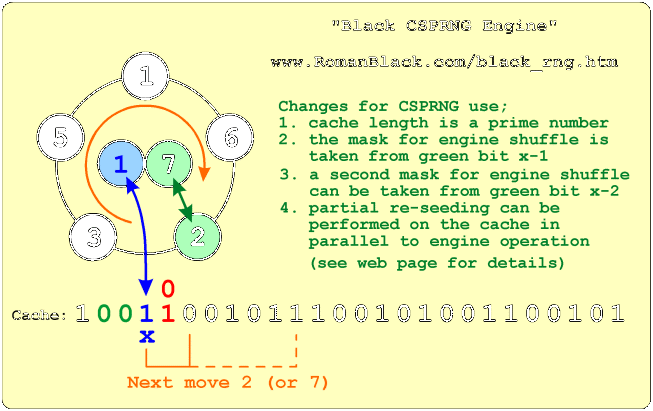- end -